# Carrier Lifetime Measurements in Semiconductors through the Microwave Photoconductivity Decay Method

Engineering
JoVE Journal
Engineering
AccessviaTrial

### Summary

As one of the important physical parameters in semiconductors, carrier lifetime is measured herein via a protocol employing the microwave photoconductivity decay method.

Asada, T., Ichikawa, Y., Kato, M. Carrier Lifetime Measurements in Semiconductors through the Microwave Photoconductivity Decay Method. J. Vis. Exp. (146), e59007, doi:10.3791/59007 (2019).

### Abstract

This work presents a protocol employing the microwave photoconductivity decay (μ-PCD) for measurement of the carrier lifetime in semiconductor materials, especially SiC. In principle, excess carriers in the semiconductor generated via excitation recombine with time and, subsequently, return to the equilibrium state. The time constant of this recombination is known as the carrier lifetime, an important parameter in semiconductor materials and devices that requires a noncontact and nondestructive measurement ideally achieved by the μ-PCD. During irradiation of a sample, a part of the microwave is reflected by the semiconductor sample. Microwave reflectance depends on the sample conductivity, which is attributed to the carriers. Therefore, the time decay of excess carriers can be observed through detection of the reflected microwave intensity, whose decay curve can be analyzed for estimation of the carrier lifetime. Results confirm the suitability of the μ-PCD protocol in measuring the carrier lifetime in semiconductor materials and devices.

### Introduction

Excess carriers in semiconductors are optically excited by the injection of photons with energy larger than the gap between the conduction and valence bands. Excited excess carriers, then, disappear by an electron–hole recombination within a time constant known as the carrier lifetime, which greatly affects the performance of semiconductor devices during operation. As one of the important parameters for semiconductor devices and materials, the carrier lifetime is very sensitive to the presence of defects in these materials, and further requires a convenient method of evaluation. J. Warman and M. Kunst developed a transient technique they named the time resolved microwave conductivity (TRMC), which involves microwave absorption to follow charge carrier dynamics in semiconductors1. Other researchers proposed the transient photo conductivity (TPC), otherwise known as the microwave photoconductivity decay (μ-PCD), which is the commonly adopted material qualification technique in semiconductor industries due to its noncontact and nondestructive measurements of the carrier lifetime. In particular, for silicon carbide (SiC), three major techniques are applicable: µ-PCD, time resolved photoluminescence (TR-PL), and time resolved free carrier absorption (TR-FCA)2,3,4,5,6,7. Among these techniques, µ-PCD is the most widely employed because compared to the other two as it exhibits surface roughness insensitivity (i.e., measurable for any given various surface roughness8,9,10) and high signal sensitivity for excited carriers (i.e., using an optimum microwave component). In general, µ-PCD has been preferred for carrier lifetime measurement in SiC and other semiconductor materials2,5,6,11,12,13,14,15,16,17,18,19.

The measurement protocol and principle of μ-PCD1,20,21 is detailed here. In principle, it uses a reflected microwave as a probe. Here, the microwave reflectance of a sample R(σ) is equivalent to the ratio between the reflected microwave intensity P(σ) and the incident microwave intensity Pin as expressed by Equation 1:(1)

By irradiation of a pulse laser, the conductivity of a sample σ changes to σ + Δσ; likewise, R (σ) transforms to R(σ + Δσ). Thus, ΔR is given by Equation 2:(2)

In a perturbation (small Δσ) approximation, R(σ + Δσ) is developed in Taylor series to yield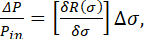(3)

while Δσ becomes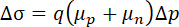, (4)

where q is the elementary charge, μp is the hole mobility, μn is the electron mobility, and Δp is the excess carrier concentration. From the preceding equations,ΔR and Δp are related by. (5)

The dependence of microwave reflectance on excess carrier concentration allows μ-PCD to observe the time decay of excess carriers, which we can use to estimate the carrier lifetime of semiconductor materials.

### Protocol

1. Preparation of the sample

1. Prepare an n-type 4H-SiC epilayer (Table of Materials).
2. Wash the sample with acetone and then with water, each for 5 min, using an
3. ultrasonic washer.
4. Use a nitrogen gun to remove moisture on the sample surface.

2. Preparation of aqueous solutions

1. Prepare 1 M each of H2SO4, HCl, Na2SO4, NaOH, or HF at 1 wt% concentration. Select and prepare an aqueous solution to be measured.
2. Prepare a quartz cell with 5 mm (length) x 20 mm (width) x 40 mm (height) dimensions and then pour the aqueous solution into it. Put the prepared sample into the cell and then immerse it into the aqueous solution.
NOTE: At least 4 mL of the aqueous solution in the quartz cell is required for the sample to be immersed entirely. When changing the solution, treat the sample with ultrasonic cleaning using acetone and pure water.

3. Preparation of the measuring equipment

1. Turn on the power supply of the 266-nm pulsed laser to excite the light source. Afterwards, set the laser mode on standby.
2. Connect the pulsed laser and an oscillator through a Bayonet Neill-Concelman (BNC) cable. Turn on the oscillator and input a 100 Hz pulse wave to the pulsed laser.
3. Connect a photodiode for trigger acquisition through a trigger input channel of the oscilloscope with a BNC cable.
4. Turn on the power supply of the photodiode.
5. Irradiate the pulsed laser and place the aperture of the microwave waveguide on the optical path of the laser light at direction normal to the light.
CAUTION: In the latter process, the experimenter should wear safety eye glasses during laser irradiation.
6. Install a half-mirror on the optical path of the pulsed laser, as shown in Figure 1, and reflect the pulsed laser to the photodiode.
7. Turn on the oscilloscope and then set its trigger threshold to a voltage sufficient to signal from the photodiode.
NOTE: The threshold value may be set smaller than the peak of the trigger signal. When an unintentional reflected light enters the photodiode, the oscilloscope displays a frequency that differs greatly from the pulsed laser frequency. In this case, repeat step 3.6.
8. Check the trigger frequency with an oscilloscope and tune the oscillator exactly.
9. Put the laser mode on standby.
10. Connect a Schottky barrier diode in a microwave waveguide for reflected microwave detection and a signal input channel of the oscilloscope, through a BNC cable.
11. Apply a 9.5 V operating voltage to a Gunn diode.
12. Place the quartz cell (step 2.2) on the stand in front of the aperture as close as possible. Fix with tape.

4. Measurement and saving data

1. Turn on the laser light oscillation and irradiate the light to the sample.
2. Place a half-waveplate (λ/2), a polarizer, and a power meter on the optical path (Figure 1).
3. Irradiate the pulsed laser to the power meter, as shown in Figure 1. Check the excitation intensity of the laser.
4. Adjust the λ/2 angle for control of the excitation intensity.
NOTE: λ/2 changes the polarization direction of the laser light, while the polarizer transmits only one light polarization direction, through which excitation intensity is controlled. Injected photon densities are set to 8 x 1013 cm−2 and for the 266-nm laser, the excitation carrier density in 4H-SiC is 4.5 x 1017 cm−3.
5. Remove the power meter from the optical path.
6. Adjust the time/div and V/div of the oscilloscope so that the peak signal is displayed on the oscilloscope.
7. Adjust the amplitude and phase of the microwave through an E–H tuner. Check the oscilloscope and look for the E–H tuner where the peak signal is at maximum. Failed adjustment of the E-H tuner results in signal loss, as depicted in Figure 2.
NOTE: An amplifier is used to strengthen the decay signal in case of insufficiently large signal relative to background noise or when it is not observed even after adjusting the E–H tuner. The amplifier is placed between the Schottky barrier diode and the signal input channel of oscilloscope with a BNC cable, as illustrated in Figure 1.
8. Repeat steps 4.6 and 4.7 to complete the tuning.
9. Adjust the time/div of the oscilloscope and sketch a decay curve in the measurement area on the oscilloscope.
10. Average the signal for an arbitrary number of times to improve the signal-to-noise ratio.
11. Save the measurement data as an electronic file to a memory and then remove it from the oscilloscope.

5. Data processing

1. Import the signal data to a personal computer.
2. Plot decay curves obtained from the experiment as a function of time.
3. Calculate the average value of background noise level, subtract it from the decay signal, and plot it as a function of time.
4. Find the peak value of the decay signal obtained in step 5.3 and then divide the decay signal by the peak value.

### Representative Results

Figure 1 shows a schematic diagram of the μ-PCD apparatus consisting of a 10 GHz microwave frequency, X waveguide band, and a rectangular waveguide. The microwave was focused by the double ridge waveguide and irradiated on the sample. The Gunn diode output power was 50 mW and the phase noise was nearly -80 dBc/Hz.

Figure 3 shows the μ-PCD decay curve of a 100-μm-thick n-type 4H-SiC sample excited on the Si-face by 266 nm in the air; μ-PCD signal (V) scaled logarithmically was the dependent variable and time (μs) was the independent variable. The signal voltage peak was approximately 0.046 V prior to amplification. Moreover, the observed voltage of the direct current (DC) component of the reflected microwave obtained from the oscilloscope DC mode was of the order of several volts. As recombination of excess carriers progressed with time, the sample’s conductivity and microwave reflectance decreased.

Figure 4 shows the normalized μ-PCD decay curve of Figure 3. Normalization enables comparison of the time constants with different peak intensities. Typically, carrier lifetime estimation based on the decay curve is carried out with the 1/e lifetime τ1/e parameter, indicating that time expended to obtain signal intensity decreases from the peak to 1/e (~0.368). Note that µ-PCD decay was not a single exponential and τ1/e was influenced by both bulk and surface recombination. However, comparing the time constant of samples having different thickness or surface condition necessitated a reference parameter. Usage of τ1/e was convenient given the good signal-to-noise ratio at the initial part of the decay curve and the simplicity of the data analysis. To characterize the µ-PCD signal, half-time lives, I40/Imax, and kD constant also adopted such parameters22,23,24. In fact, τ1/e was adopted in the SEMI standard: SEMI MF 15358 as the standard for carrier lifetime measurement of Si. For the decay curve in Figure 4, τ1/e was approximately 0.34 μs.

In Figure 5, the quartz cell, containing the aqueous solution and with the sample on its wall, was placed on the stand in front of the aperture11. Each intensity of the irradiated microwave and the reflected microwave from the sample, as well as the μ-PCD signal-to-noise ratio, were dependent on the distance between the sample and the aperture, which, ideally, should be as close as possible. In the actual measurement, the distance obtained was as close as possible; measurement using the quartz cell yielded a distance of 0.5 mm, which was of the same as the thickness of the quartz cell glass.

Figure 6 shows μ-PCD decay curves of the n-type 4H-SiC in the air and in aqueous solutions. An excitation light of 266 nm was irradiated to the Si-face of the 4H-SiC. Aqueous solutions used had concentrations, as mentioned previously, as follows: 1 M each of H2SO4, HCl, Na2SO4, or NaOH, or 1 wt% of HF. The time constant of the decay curves was longer with the sample immersed into the acidic aqueous solutions (i.e., H2SO4, HCl, or HF), implying that acidic solutions passivated surface states on the Si-face and reduced surface recombination of the excess carriers.

Figure 7 shows the pH dependence of τ1/e of the n-type 4H-SiC sample excited on the Si-face at 266 nm of light. The pH was calculated from the molar concentrations of H2SO4, HCl, and NaOH. This figure indicated the carrier lifetime dependence on pH aqueous solutions; therefore, lower pH would have more effects on the carrier lifetime.

Surface recombination velocity S was calculated to reproduce the τ1/e used for the samples. The decay model of excess carriers has been reported in refs. 2 and 3. To obtain the excess carrier concentration Dn(x, t), the following continuity equation was solved. Here, Dn(x, t) was defined as a function of time t and depth x in a semiconductor layer; thus,,              (6)

where τB is bulk lifetime due to the Shockley–Read–Hall (SRH) recombination, Da is the ambipolar diffusion coefficient, B is the radiation recombination coefficient, and C is the Auger recombination coefficient.

At the excited and other surfaces, boundary conditions were given by Equation 7: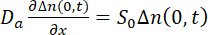and(7)

where S0 and SW denote the surface recombination velocity of the excited and other surfaces, respectively, and W is the layer thickness.

Moreover, the initial excess carrier concentration profile using light pulse illumination could be expressed using Equation 8:(8)

where g0 is carrier concentration at x = 0 and a is the absorption coefficient.

Solving Equation 6 by employing the boundary conditions of Equation 7 and the initial condition of Equation 8 provided the excess carrier decay curves. In the process, S was estimated by comparing the τ1/e obtained from the experiments and from the calculated decay curves. Least squares fitting minimized errors between the experimental τ1/e in the all conditions and the calculated τ1/e with parameters S0, Sw, and τB.

As depicted in Equation 6 carrier recombination is the summation of various decay components, namely, surface, SRH, radiative, and Auger recombinations, the last two having remarkable high carrier density. On the other hand, SRH recombination depends on point defects and dislocations in the bulk of the semiconductor material that form energy levels in the semiconductor band gap. The energy levels act as stepping stones for carrier transition between the valence and conduction bands.

μ-PCD also shows nonlinearity at a high injection condition, and overestimates carrier lifetime13,25,26. Figure 8 shows the measured μ-PCD under a high excitation condition. Note that the decay curve for an injected photon density of 1015 cm−2 became more gradual compared to that for photon density of 1014 cm−2, owing to microwave nonlinearity. Furthermore, the measurement examples shown in Figure 3, Figure 4, and Figure 6 were obtained for an injected photon density of 8 x 1013 cm−2 resulting in negligible microwave nonlinearity, and Auger and radiative recombinations but dominant SRH and surface recombinations.

Figure 6 can be taken to exemplify decay curve calculations for the n-type 4H-SiC Si-face excited by 266 nm light, by referring to the dashed lines, where τB = 3 μs and S for the Si-face SSi = 200 cm/s or 700 cm/s. For both SSi settings, the experimental decay curve measured in neutral pH (air, 1 M Na2SO4) and in the acidic condition (1 M H2SO4), respectively, were well reproduced, which meant that SSi for the n-type 4H-SiC signiﬁcantly reduced from 700 cm/s to 200 cm/s in acidic aqueous solutions as hydrogen passivated the surface states on the Si-face.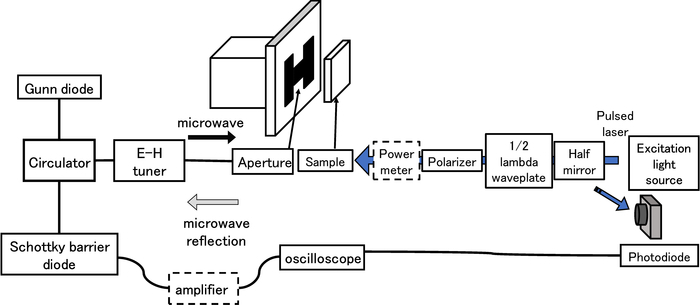Figure 1: Schematic diagram of the μ-PCD device. A part of the laser light is reflected by the half-reflection mirror. The reflected laser is detected by the photodiode, and a signal coming from the photodiode is used as a trigger for the oscilloscope. A microwave is generated from the Gunn diode in the direction bent by the circulator; then, a microwave goes through the aperture and irradiates the sample. The reflected microwave from the sample comes back to the aperture and into the circulator, where it is detected by the Schottky barrier diode. Finally, the signal coming from the Schottky barrier diode is observed by the oscilloscope. Please click here to view a larger version of this figure.Figure 2: The μ-PCD signal for a failed tuning of E-H tuner. No measurable peak is observed for a failed tuning. Please click here to view a larger version of this figure.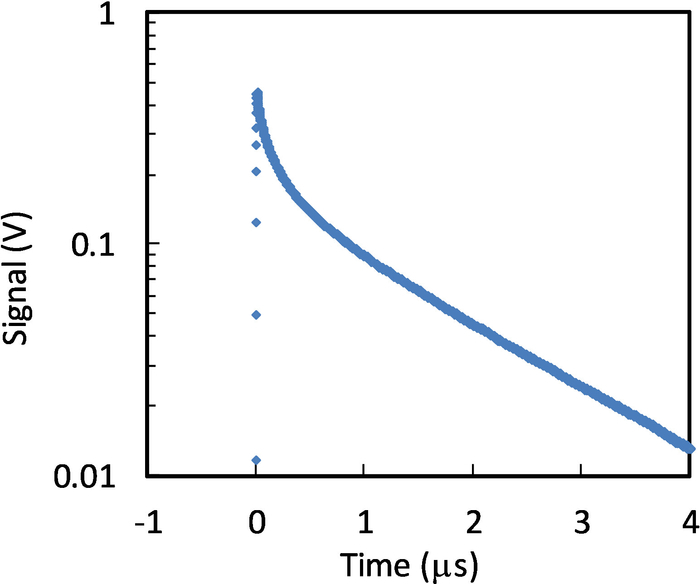Figure 3: The μ-PCD decay curve for the n-type 4H-SiC sample with excitation on the Si-face by 266 nm in air. The pulsed laser is irradiated at time = 0 s at which the signal intensity is at maximum. This figure has been modified from Ichikawa et al.11 with permissions. Please click here to view a larger version of this figure.Figure 4: Normalized μ-PCD decay curve for the n-type 4H-SiC sample with excitation on the Si-face by 266 nm in air. The maximum value of the decay curve in Figure 2 is normalized to unity. The value of the dashed line is 1/e, and τ1/e is approximately 0.34 μs as depicted. This figure has been modified from Ichikawa et al.11 with permissions. Please click here to view a larger version of this figure.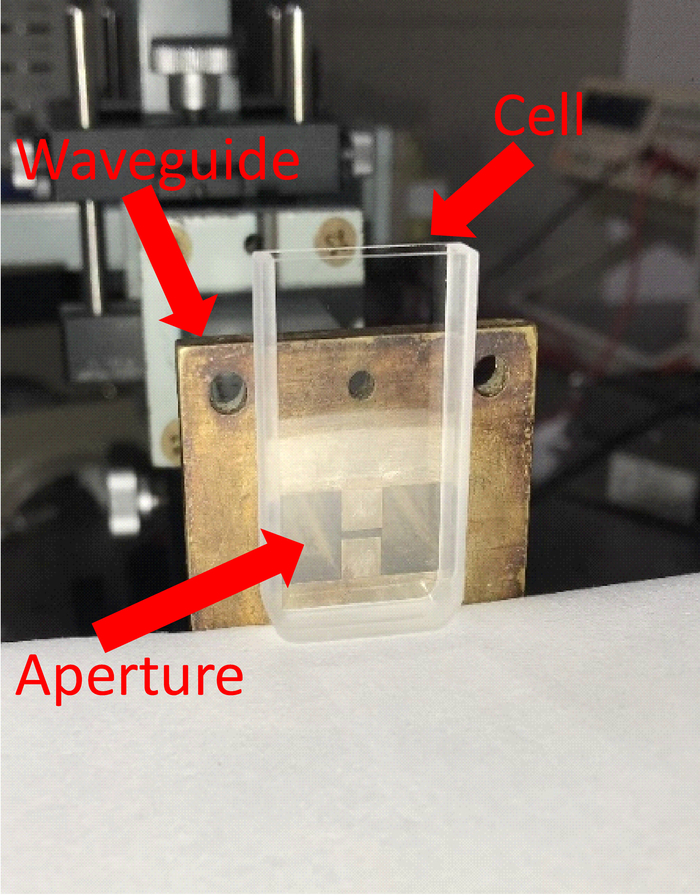Figure 5: Image of μ-PCD measurement in an aqueous solution in a quartz cell. The quartz cell is placed on the stand in front of the aperture to allow μ-PCD decay curve measurement in an aqueous solution. The cell dimension is 5 mm x 20 mm x 40 mm (length x width x height). Please click here to view a larger version of this figure.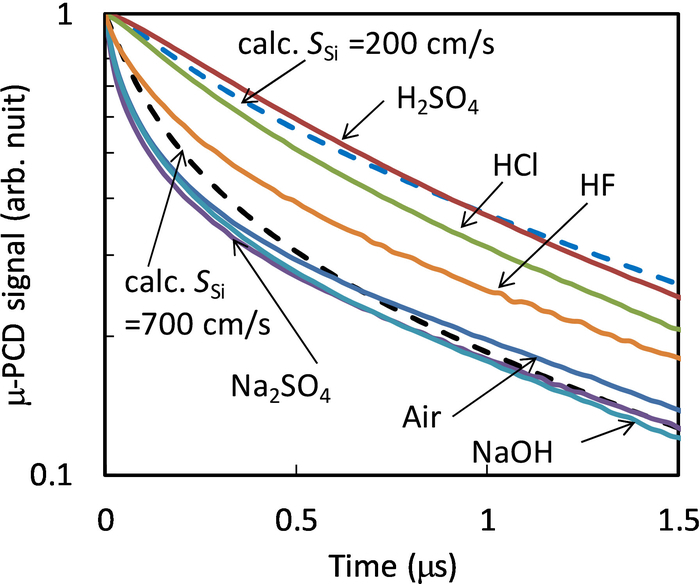Figure 6: Normalized and calculated μ-PCD decay curves for the n-type 4H-SiC sample with excitation on the Si-face by 266 nm, in air and aqueous solutions. Solid lines represent the μ-PCD experimental result curves for the aqueous solutions of H2O, H2SO4, HCL, Na2SO4, NaOH, or HF. The dashed lines are calculated curves with the bulk carrier lifetime in the epilayers, τB = 3 μs, and the surface recombination velocity for the Si-face, SSi = 200 cm/s or 700 cm/s. This figure has been modified from Ichikawa et al.11 with permissions. Please click here to view a larger version of this figure.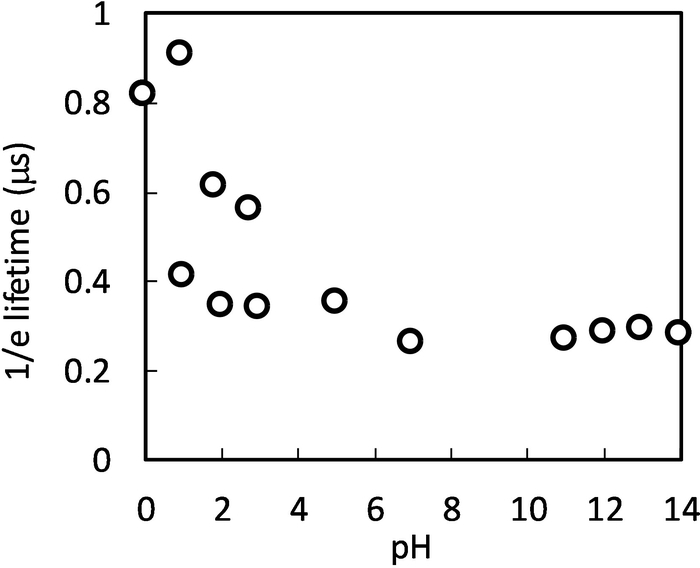Figure 7: The pH dependence of τ1/e for the n-type 4H-SiC sample with excitation on the Si-face by 266 nm. Carrier lifetime increases as the pH of the aqueous solution decreases. This result indicates that lower pH will have more effects on the carrier lifetime. This figure has been modified from Ichikawa et al.11 with permissions. Please click here to view a larger version of this figure.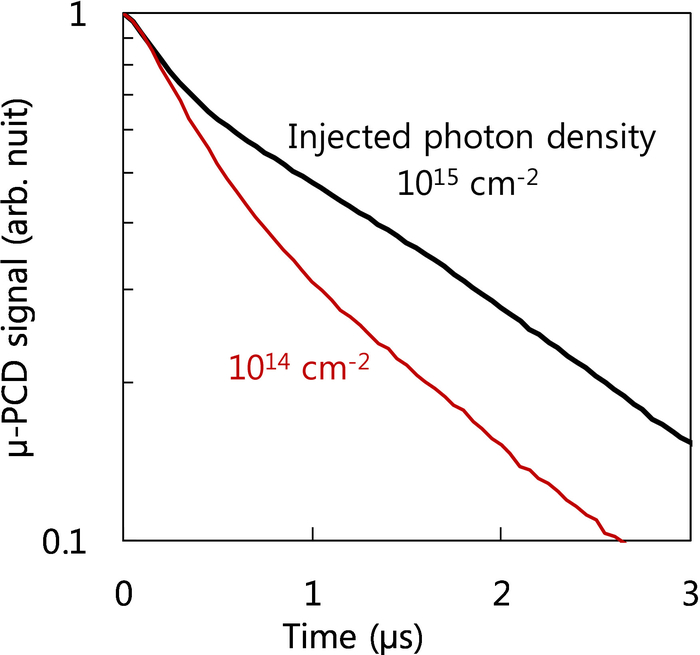Figure 8: The μ-PCD decay curve of n-type 4H-SiC with excitation of injected photon density of 1014 or 1015 cm−2 on the Si-face by 266 nm. Measurement with high excitation at photon density of 1015 cm−2 makes a more gradual decay curve than that with lower photon density due to nonlinearity of microwave reflectivity. Please click here to view a larger version of this figure.

### Discussion

In the μ-PCD protocol, step 4.7 is the most important point. The E–H tuner was incorporated with a movable short circuit in the E and H planes, respectively. Thus, moving the short circuit of the E tuner or the H tuner changes the amplitude and phase of the reflected microwave and maximizes the signal amplitude. Tuning has a large influence on the waveform of the decay curve and must be performed strictly. In case of a weak signal strength where tuning might be difficult, a few tens of tuning averages may be used. For failed tuning, the μ-PCD decay curves are not observable; only the noise signal of an oscilloscope is observed. Figure 2 shows the oscilloscope waveform in such a case.

It is easy to measure highly resistive samples as there is no lower conductivity limit. When the sample resistivity is low or when the sample is thick, the skin effect of the microwave is not negligible. The distance until the electric field intensity of the microwave becomes 1/e times is referred to as skin depth, which is expressed by Equation 9:(9)

where ω is the angular frequency of the microwave, and ε, ρ, and μ represent the sample’s dielectric constant, resistivity, and permeability, respectively. In the case of Si and SiC, approximate δ values for the 10 GHz microwave were 9 mm at 50 Ω∙cm, 2 mm at 10 Ω∙cm, 500 μm at 1 Ω∙cm, and 150 μm at 0.1 Ω∙cm. Therefore, measurements for samples with typical thicknesses (several hundred micron) at less than 0.1 Ω∙cm will lose δ accuracy. On the other hand, the microwave and optical radiation are incident from the opposite of the wafer in this protocol. A negligible skin effect indicates better microwave and optical radiation from the same side.

Lower limits depend on the resistivity and thickness of the sample resulting from its interaction with the microwave. For highly resistive samples, the typical lower limits of the excess carriers are on the order of 1012 cm−3. On the other hand, electron–hole scattering must be considered at excess carriers greater than 1016 cm−3, as discussed in ref. 13.

The μ-PCD decay curves became gentle at high excitation density due to unproportionality of the microwave reflectivity to the excess carrier concentration such that Equation (3) would lose its validity13,25,26 and τ1/e would be overestimated. Figure 8 shows the μ-PCD decay curve of a chemical mechanical polishing surface treatment n-type 4H-SiC with excitation on the Si-face by 266 nm under high excitation intensity.

Moreover, time resolution depends on the performance of the measurement apparatus such as an excitation source, an oscilloscope, and an amplifier. For example, in this study, the apparatus consisted of a pulsed laser with pulse width of 1 ns as the excitation source and an oscilloscope having a frequency band of 500 MHz. Consequently, the minimum measurable lifetime was estimated at 2 ns.

As mentioned earlier, μ-PCD is very useful for characterization of semiconductors such as Si. Nevertheless, its application can be extended to other materials, for instance, in photoactive materials including TiO227,28,29,30.

Furthermore, aside from the μ-PCD, TR-PL2 and TR-FCA introduced at the earlier sections are the other two carrier lifetime measurement techniques. TR-PL observes the time change of photoluminescence caused by carrier recombination while TR-FCA observes the time change of probe light absorption4. Specifically, free carrier absorption occurs when light with energy smaller than the band gap is irradiated during carrier excitation3. Nevertheless, compared to these two, μ-PCD directly observes electrical conductivity by microwave and has a high surface roughness and signal sensitivity, making it the more ideal method for carrier lifetime measurement for semiconductor device applications.

### Disclosures

The authors have nothing to disclose.

### Acknowledgments

This work was supported by the Nagoya Institute of Technology, Japan.

### Materials

 Name Company Catalog Number Comments n-type 4H-SiC epilayer Ascatron AB http://ascatron.com/ Sample 266 nm pulsed laser CryLaS GmbH http://www.crylas.de/ FQSS 266-50 Excitation light source Photodiode THORLABS https://www.thorlabs.com/index.cfm DET10A/M Trigger signal detection Schottky barrier diode ASI http://www.advancedsemiconductor.com/ 1N23WE Reflected microwave detection Gun diode Microsemi https://www.microsemi.com/ MO86751C Microwave generation source E-H tuner SPC ELECTRONICS CORPORATION http://www.spc.co.jp/index.html microwave component Circulator SPC ELECTRONICS CORPORATION http://www.spc.co.jp/index.html microwave component Rectangular waveguide SPC ELECTRONICS CORPORATION http://www.spc.co.jp/index.html microwave component Double ridge waveguide SPC ELECTRONICS CORPORATION http://www.spc.co.jp/index.html microwave component Crystal mount SPC ELECTRONICS CORPORATION http://www.spc.co.jp/index.html microwave component Acetone KANTO CHEMICAL CO.,INC. https://www.kanto.co.jp/ GE00001 Sample cleaning Sulfuric acid KANTO CHEMICAL CO.,INC. https://www.kanto.co.jp/ GE00257 Acidic aqueous solution Hydrochloric acid KANTO CHEMICAL CO.,INC. https://www.kanto.co.jp/ GE00238 Acidic aqueous solution Hydrogen fluoride KANTO CHEMICAL CO.,INC. https://www.kanto.co.jp/ 18083-1B Acidic aqueous solution Sodium hydroxide KANTO CHEMICAL CO.,INC. https://www.kanto.co.jp/ 37184-00 Alkaline aqueous solution Sodium sulfate KANTO CHEMICAL CO.,INC. https://www.kanto.co.jp/ 37280-00 Neutral aqueous solution